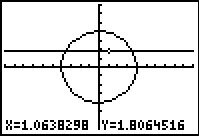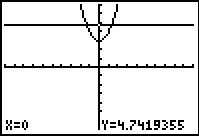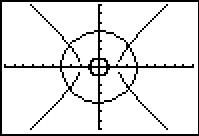••• ##### Device
• TI-83 Plus Family
• TI-84 Plus
• TI-84 Plus Silver Edition
•TI-84 Plus C Silver Edition
•TI-84 Plus CE

# Precalculus: Nonlinear Systems of Equations

by Texas Instruments#### Overview

Students see the many ways that certain types of graphs (linear/quadratic and quadratic/quadratic) can intersect each other and how many potential intersection points are possible.

#### Key Steps

•In problem 1, students explore the number of possible intersection points when using nonlinear system of equations. They begin by graphing a circle (shown to the right), a horizontal and vertical line.

•Students move on to a parabola and investigate its possible intersection points. Students will see that for graphs of a linear and quadratic function, there are either 0, 1, or 2 points of intersection.

Students continue to problem 1 by investigating an ellipse and a hyperbola.

•Students use the graphs of a hyperbola and two circles to determine the number of intersection points. They will see that the inside circle is a circle with radius one and has no points of intersection. The larger circle has four points of intersection.